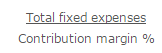# Is Depreciation a Fixed Cost or Variable Cost?

Depreciation is a fixed cost, because it recurs in the same amount per period throughout the useful life of an asset. Depreciation cannot be considered a variable cost, since it does not vary with activity volume.  However, there is an exception. If a business employs a usage-based depreciation methodology, then depreciation will be incurred in a pattern that is more consistent with a variable expense.

Depreciation is a method of allocating the cost of a tangible asset over its useful life. Businesses depreciate long-term assets for both tax and accounting purposes. It decrease in an asset’s value caused by unfavorable market conditions.

For example, a logging machine is depreciated based on the number of hours that it is used, so that depreciation expense will vary with the number of trees cut. If these trees are then sold to generate revenue, then it can be said that the related depreciation behaves more like a variable cost than a fixed cost. However, usage-based depreciation systems are not commonly used, so in most cases depreciation cannot be considered a variable cost.

If depreciation is considered a fixed cost, then it is included in the numerator of the formula used to calculate the break even sales of a business, which is:If it is considered a variable cost, for which a case can be argued if usage-based depreciation is employed, then it is instead used to reduce the amount of the contribution margin percentage in the denominator of the equation.